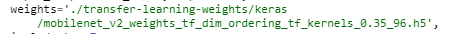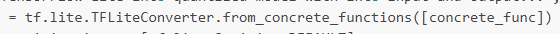# Keras pretrain weight

may I get this weight please？thanksHi @LenBonJJ, the models are now here: http://cdn.edgeimpulse.com/transfer-learning-weights. Just append the `/keras/mobilenet` etc part there.

We’re fixing this next week in the notebooks.

may I know your quantization way？
I am trying to quantized and used in openmv but failed
and this is my quantization code and error:

I will appreciate it if you can give me some adviceby the way, I used this pretrain model:“mobilenet_v2_weights_tf_dim_ordering_tf_kernels_0.35_96.h5”

@LenBonJJ this is the code we use:

``````        print('Converting TensorFlow Lite int8 quantized model with int8 input and output...', flush=True)
converter_quantize = tf.lite.TFLiteConverter.from_concrete_functions([concrete_func])
converter_quantize.optimizations = [tf.lite.Optimize.DEFAULT]
converter_quantize.representative_dataset = dataset_generator
# Force the input and output to be int8
converter_quantize.target_spec.supported_ops = [tf.lite.OpsSet.TFLITE_BUILTINS_INT8]
converter_quantize.inference_input_type = tf.int8
converter_quantize.inference_output_type = tf.int8
tflite_quant_model = converter_quantize.convert()
open(os.path.join(dir_path, filename), 'wb').write(tflite_quant_model)
return tflite_quant_model
``````

Not sure what’s going on with your model, but hard to give support outside of the Studio (where it just works)

oh thanks
I think I know where the problem ismay I know how can you get the concrete_func?
Thank you very much!Hi @LenBonJJ it’s actually part of TensorFlow. See https://github.com/tensorflow/tensorflow/blob/08756627a6defd3e4131ec8af5e81009f1de1f0d/tensorflow/lite/python/lite.py#L1279